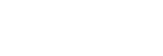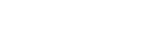Difference Amp
The first question one may have is, “Can’t I build an instrumentation amplifier out of simple op amps?” The short answer is yes, you can. But there are always trade-offs! One may first think of a simple difference amplifier circuit (Figure 1), sometimes called a subtractor.

This is a very simple circuit that provides for differential gain and has some common-mode rejection, which is exactly what an INA is intended to do. Earlier, I mentioned trade-offs, and this circuit has a couple. First of all, let’s look at the input impedance. It is relatively low, as determined by the values of the resistors, which may be on the order of 100 kΩ. Secondly, the input impedances aren’t matched, meaning a different current will flow through each leg, causing the common-mode rejection to suffer. The other shortcoming of this simple circuit is the need for resistor matching. The common-mode rejection of this circuit is predominately determined by the level of matching within the resistor pairs, not the op amp itself. Any mismatch in these resistor pairs will reduce the common-mode rejection. The common-mode rejection of this difference amplifier can be calculated as:Where: Rt = total mismatch of the resistor pairs in fractional form

For example, assume R1 = R2 = R3 = R4 (providing unity gain), and the resistor mismatch is 1%. Using the above equation:CMR = 46 dB

As this example shows, the performance one can achieve with this simple circuit is extremely limited. Even when matching resistors by hand, it will be difficult to achieve a common-mode rejection any greater than 66 dB. In addition, this does not take into account fluctuations due to temperature, as any difference in temperature coefficients among the resistors will further increase the mismatch and result in worse common-mode rejection. Taking all of these factors and limitations into account, a monolithic difference amplifier is usually the best solution for relatively high-performance applications.

The difference-amplifier circuit discussed previously is technically not an instrumentation amplifier, but is useful for certain applications requiring high speed and/or high common-mode voltage levels. For precision applications, an actual instrumentation amplifier is often the best choice. There are two common circuits utilized to create an instrumentation amplifier, one based on two amplifiers and one based on three amplifiers. Both will be discussed in detail. Note that these basic circuits can be constructed using standard op amps, but are also the underlying circuit concepts used in many of the monolithic instrumentation amplifiers offered today.

Two Op Amp INA